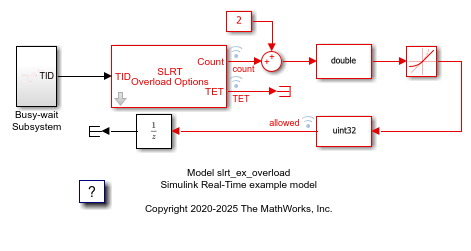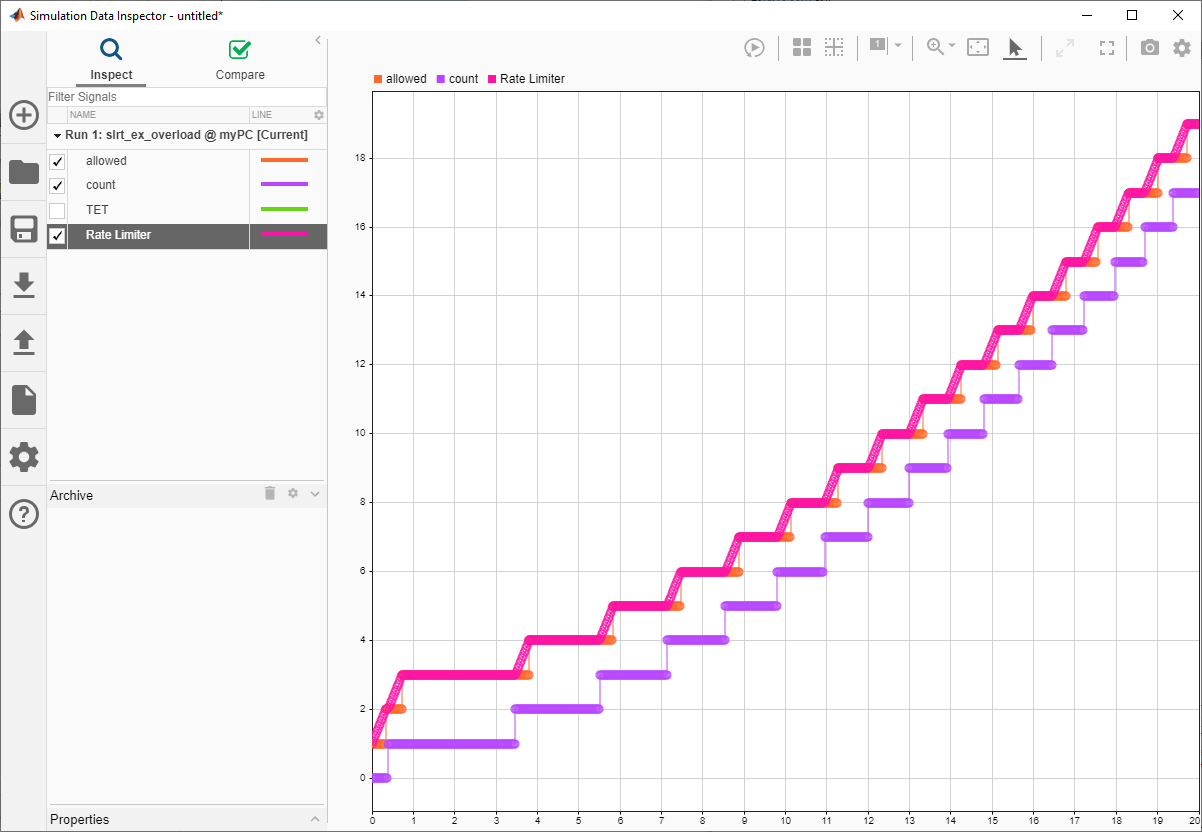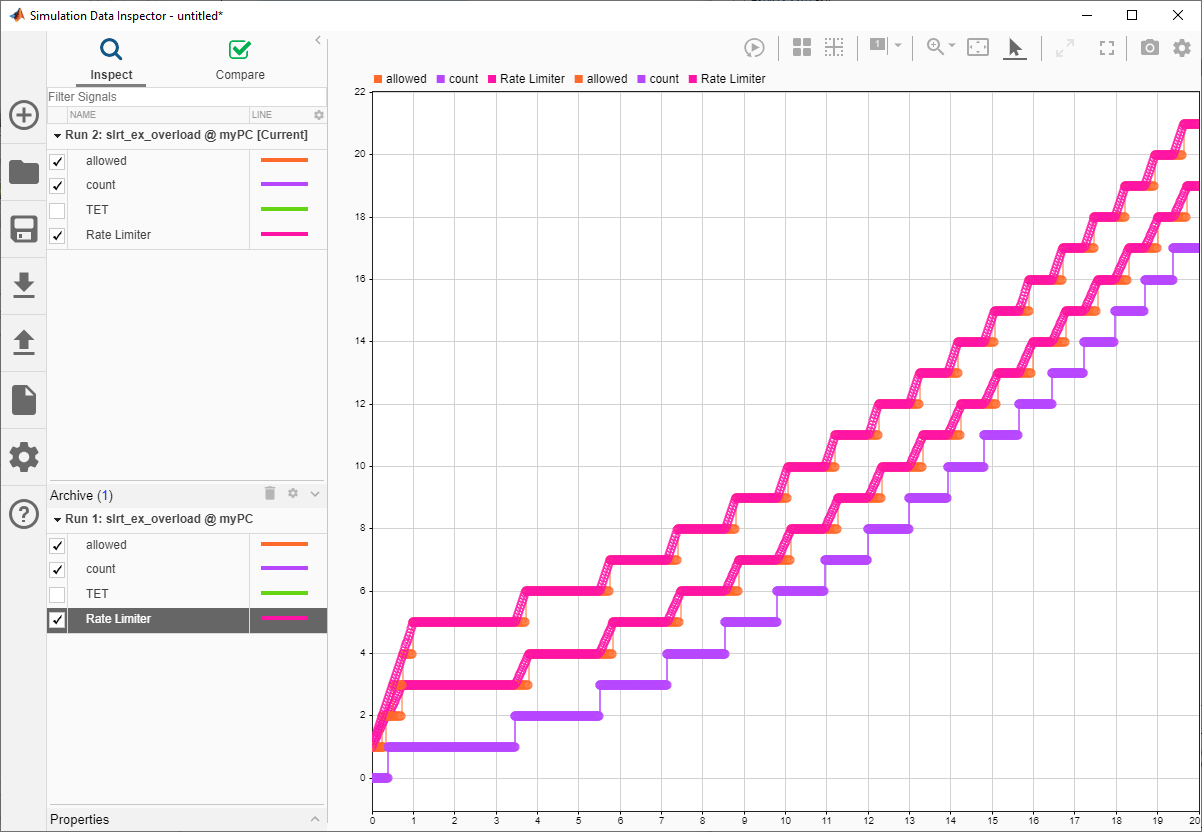The SLRT Overload Options block outputs the current CPU overload count for the identified sample rate.

This example shows how to design a model that uses the SLRT Overload Options block to monitor the rate at which CPU overloads occur. The rate of CPU overloads information can be useful when tuning performance of a model for which a low CPU overload rate is acceptable.

### Open, Build, and Run the Model

In the MATLAB Command Window, type:

```open_system(fullfile(matlabroot, 'toolbox','slrealtime','examples','slrt_ex_overload')); ```Name the signal coming out from the outport of rate limiter block as Rate Limiter and log it in the Simulation Data Inspector.

```p = get_param('slrt_ex_overload/Rate Limiter','PortHandles'); l = get_param(p.Outport,'Line'); set_param(l,'Name','Rate Limiter'); Simulink.sdi.markSignalForStreaming('slrt_ex_overload/Rate Limiter',1,'on'); ```

Build the model.

```model = 'slrt_ex_overload'; set_param(model, 'RTWVerbose', 'off'); evalc('slbuild(model)'); ```

```tg = slrealtime; connect(tg); load(tg,model); start(tg); pause(20); stop(tg); ```

### Open Simulation Data Inspector

To view the rate at which CPU overloads occur, open the Simulation Data Inspector.

In the MATLAB Command Window, type:

```Simulink.sdi.view; ```

### Examine CPU Overload Rate Data

In the Simulation Data Inspector, the graph shows:

• Bottom rising stair step signal -- This signal indicates the number of CPU overloads that occurred.

• Top rising stair step signal -- This signal indicates the number of CPU overloads that are allowed, which is (occurred + 2).

• Rising slew rate -- This signal indicates the rate at which CPU overloads occur. When the rising slew rate becomes greater than the top rising stair step signal, the rate of CPU overloads is greater than are allowed.### Modify Rate of CPU Overloads

To modify the rate at which CPU overloads occur in the model, modify the `Constant2` parameter value.

### Modify Allowed Rate of CPU Overloads

To modify the rate of CPU overloads that are acceptable in the model, modify the `RisingSlowLimit` parameter value.

### Build and Run Model with Changed Overload Rates

In the MATLAB Command Window, type:

```load(tg,model); ```

To modify the rate of CPU overloads that are acceptable in the model

```tg.setparam('slrt_ex_overload/Rate Limiter','RisingSlewLimit',0.004); ```

To modify the rate at which CPU overloads occur in the model

```tg.setparam('slrt_ex_overload/Constant2','Value',4); ```

run the modified application on the target computer

```start(tg); pause(20); stop(tg); ```

In the Simulation Data Inspector, compare the signal data from the simulation runs and observe the change to the CPU overload rate.```bdclose('all'); ```